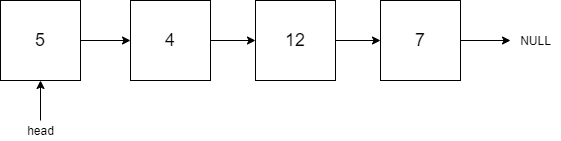# Search in a Linked List

Posted: 10 Dec, 2020
Difficulty: Easy

## PROBLEM STATEMENT

#### You are given a Singly Linked List of integers with a head pointer. Every node of the Linked List has a value written on it.#### Now you have been given an integer value 'K'. Your task is to check whether a node having a value equal to 'K' exists in the given linked list or not.

##### Input Format:
``````The first line of the input contains an integer 'T' representing the number of test cases or queries to be processed. Then the 'T' test case follows.
The first line of each test case contains space-separated integers denoting the values of nodes of the Linked List. The Linked List is terminated with -1. Hence, -1 is never a node value of the Linked List.
The third line of each test case contains a single integer 'K' which is desired to be checked in the Linked List.
For more clarity, please refer to the sample inputs.
``````
##### Output Format:
``````For each test case, print 1 if the desired value 'K' exists in the Linked List; otherwise, print 0.
Print the answer for each test case in a new line.
``````
##### Note:
``````You do not need to print anything, and it has already been taken care of. Just implement the given function.
``````
##### Constraints:
``````1 <= T <= 10
1 <= L <= 10^5
1 <= data <= 10^9 and data != -1
1 <= K <= 10^9

Where 'T' represents the number of test cases,  'L' represents the total number of nodes in the Linked List, "data" represents the value at each node, and 'K' is the given integer.
Time Limit: 1 sec.
``````Approach 1

Let's try to build a recursive solution to this problem

The recursive function has three cases:

1. The Head is NULL(it means that we have reached the end of the linked list without finding value ‘K’ that means the particular value is not present in the Linked List. So, in this case, we will return 0 as our answer).
2. The Head is Not NULL, and the value of the head is equal to the desired value ‘K’ we will return 1 in this case as we found the desired value in the Linked List.
3. The Head is Not NULL, and the current value is not equal to the desired value ‘K’, so it will recur to the next node of the head and undergo the same process there.